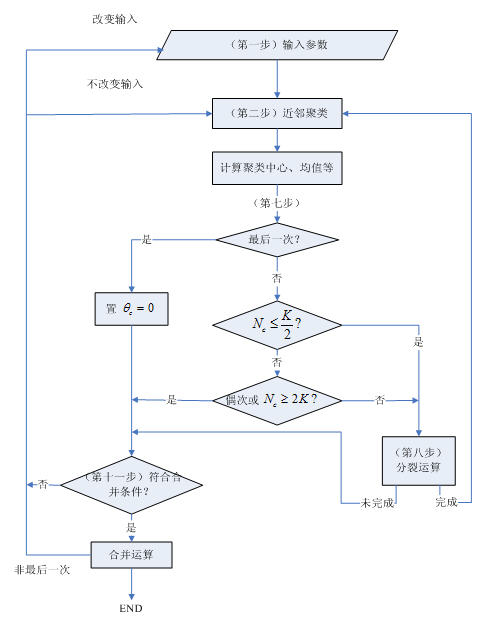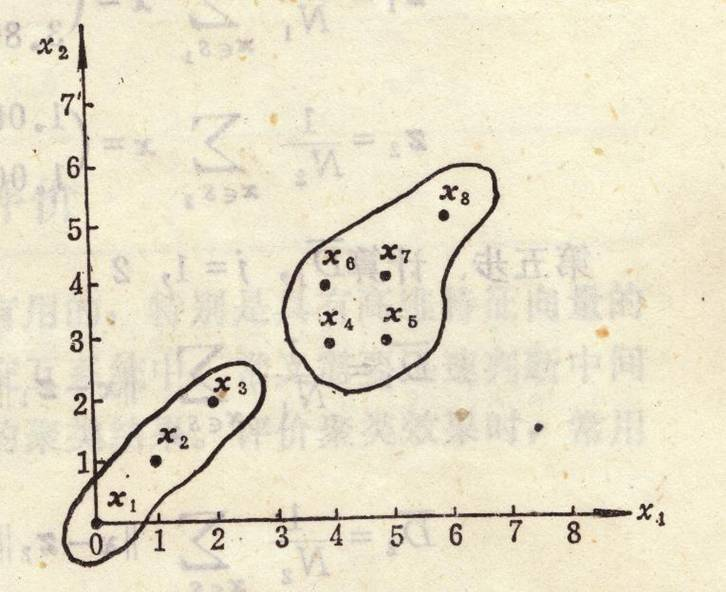# 聚类算法：ISODATA算法

1. 与K-均值算法的比较

–K-均值算法通常适合于分类数目已知的聚类，而ISODATA算法则更加灵活；

–从算法角度看， ISODATA算法与K-均值算法相似，聚类中心都是通过样本均值的迭代运算来决定的；

–ISODATA算法加入了一些试探步骤，并且可以结合成人机交互的结构，使其能利用中间结果所取得的经验更好地进行分类。

2. ISODATA算法基本步骤和思路

（1）  选择某些初始值。可选不同的参数指标，也可在迭代过程中人为修改，以将N个模式样本按指标分配到各个聚类中心中去。

（2）  计算各类中诸样本的距离指标函数。

（3）~（5）按给定的要求，将前一次获得的聚类集进行分裂和合并处理（（4）为分裂处理，（5）为合并处理），从而获得新的聚类中心。

（6）  重新进行迭代运算，计算各项指标，判断聚类结果是否符合要求。经过多次迭代后，若结果收敛，则运算结束。

3. ISODATA算法流程图：4.ISODATA算法

预选NcNc个初始聚类中心{z1,z2,…zNc}{z1,z2,…zNc}，它可以不等于所要求的聚类中心的数目，其初始位置可以从样本中任意选取。

预选：KK  = 预期的聚类中心数目；

θNθN = 每一聚类域中最少的样本数目，若少于此数即不作为一个独立的聚类；

θSθS = 一个聚类域中样本距离分布的标准差；

θcθc= 两个聚类中心间的最小距离，若小于此数，两个聚类需进行合并；

LL= 在一次迭代运算中可以合并的聚类中心的最多对数；

II  = 迭代运算的次数。

，即||x−zj||||x−zj||的距离最小，则x∈Sjx∈Sj。

（以上各步对应基本步骤（1））

zj=1Nj∑x∈Sjx,j=1,2,⋯,Nczj=1Nj∑x∈Sjx,j=1,2,⋯,Nc

D¯j=1Nj∑x∈Sj∥x−zj∥,j=1,2,⋯,NcD¯j=1Nj∑x∈Sj‖x−zj‖,j=1,2,⋯,Nc

D¯=1N∑j=1NNjD¯jD¯=1N∑j=1NNjD¯j

（以上各步对应基本步骤（2））

1. 若迭代运算次数已达到I次，即最后一次迭代，则置θc =0，转至第十一步。
2. 若Nc≤K2Nc≤K2
，即聚类中心的数目小于或等于规定值的一半，则转至第八步，对已有聚类进行分裂处理。
3. 若迭代运算的次数是偶数次，或Nc≥2KNc≥2K
，不进行分裂处理，转至第十一步；否则（即既不是偶数次迭代，又不满足Nc≥2KNc≥2K），转至第八步，进行分裂处理。

（以上对应基本步骤（3））

σj=(σ1j,σ2j,…,σnj)Tσj=(σ1j,σ2j,…,σnj)T

其中向量的各个分量为

σij=1Nj∑k=1Nj(xik−zij)2−−−−−−−−−−−−−−−⎷σij=1Nj∑k=1Nj(xik−zij)2

式中，i = 1, 2, …, n为样本特征向量的维数，j = 1, 2, …, Nc为聚类数，Nj为Sj中的样本个数。

1. D¯j>D¯D¯j>D¯和Nj > 2(θN + 1)，即Sj中样本总数超过规定值一倍以上，
2. Nc≤K2Nc≤K2

则将zj 分裂为两个新的聚类中心和，且Nc加1。 中对应于σjmax的分量加上kσjmax，其中；中对应于σjmax的分量减去kσjmax。

如果本步骤完成了分裂运算，则转至第二步，否则继续。

（以上对应基本步骤（4）进行分裂处理）

Dij=||zi−zj||，i=1,2,…,Nc−1，j=i+1,…,NcDij=||zi−zj||，i=1,2,…,Nc−1，j=i+1,…,Nc

{Di1j1,Di2j2,…,DiLjL}{Di1j1,Di2j2,…,DiLjL}

式中Di1j1<Di2j2<…<DiLjLDi1j1<Di2j2<…<DiLjL。

z∗k=1Nik+Njk[Nikzik+Njkzjk],k=1,2,⋯,Lzk∗=1Nik+Njk[Nikzik+Njkzjk],k=1,2,⋯,L

式中，被合并的两个聚类中心向量分别以其聚类域内的样本数加权，使Z∗kZk∗为真正的平均向量。

（以上对应基本步骤（5）进行合并处理）

在本步运算中，迭代运算的次数每次应加1。

[算法结束]

5.例子：试用ISODATA算法对如下模式分布进行聚类分析：

{x1(0,0),x2(3,8),x3(2,2),x4(1,1),x5(5,3),x6(4,8),x7(6,3),x8(5,4),x9(6,4),x10(7,5)}{x1(0,0),x2(3,8),x3(2,2),x4(1,1),x5(5,3),x6(4,8),x7(6,3),x8(5,4),x9(6,4),x10(7,5)}（1）   设置控制参数

取K=3，θN=1，θS=1，θc=4，L=1，I=4

（2）   按最小距离原则将模式集（xi）中每个模式分到某一类中。

由于此时只有一个聚类中心，因此S1={x1, x2, …, x10}，N1=10

（3）   因N1>θN ，无子集可抛

（4）   修改聚类中心

z1=1N1∑x∈S1x=(3.93.8)z1=1N1∑x∈S1x=(3.93.8)

（5）   计算模式样本与聚类中心间的平均距离D¯1D¯1

D¯1=1N1∑x∈S1∥x−z1∥=3.0749D¯1=1N1∑x∈S1‖x−z1‖=3.0749

（6）   计算全部模式样本和其对应聚类中心的总平均距离

D¯=D¯1=3.0749D¯=D¯1=3.0749

（7）   因不是最后一次迭代，且Nc<K/2Nc<K/2，进入（8）

（8）   计算S1中的标准差向量

σ1=(2.21132.5219)σ1=(2.21132.5219)

（9） σ1maxσ1max  中的最大分量是2.5219，因此 σ1max=2.5219σ1max=2.5219。

（10）因σ1max>θsσ1max>θs 且Nc<K2Nc<K2，可将z1分裂成两个新的聚          类。设rj=0.5σ1max≈1.261rj=0.5σ1max≈1.261.则

z+1=(3.95.061),z−1=(3.92.539)z1+=(3.95.061),z1−=(3.92.539)

为方便起见，将z+1z1+和z−1z1−表示为z1和z2，Nc加1 ，Nc=2Nc=2.

（11）   重新进行分类

 样本点 特征值 到z1的距离 到z2的距离 聚类结果 X1 0 0 6.3893 4.6537 S2 X2 3 8 3.0737 5.5347 S1 X3 2 2 3.6027 1.975 S2 X4 1 1 4.9902 3.2831 S2 X5 5 3 2.3362 1.1927 S2 X6 4 8 2.9407 5.4619 S1 X7 6 3 2.9424 2.15 S2 X8 5 4 1.5283 1.8288 S1 X9 6 4 2.3528 2.5582 S1 X10 7 5 3.1006 3.9581 S1

S1={x2,x6,x8,x9,x10},N1=5S1={x2,x6,x8,x9,x10},N1=5

S2={x1,x3,x4,x5,x7},N2=5S2={x1,x3,x4,x5,x7},N2=5

（12）   因N1>θN 且N2>θN，无子集可抛。

（13）   修改聚类中心

z1=1N1∑x∈S1x=(55.8)z1=1N1∑x∈S1x=(55.8)

z2=1N2∑x∈S2x=(2.81.8)z2=1N2∑x∈S2x=(2.81.8)

（14）   计算模式样本与聚类中心间的平均距离D¯j,j=1,2D¯j,j=1,2

D¯1=1N1∑x∈S1∥x−z1∥=2.2806D¯1=1N1∑x∈S1‖x−z1‖=2.2806

D¯2=1N2∑x∈S2∥x−z2∥=2.4093D¯2=1N2∑x∈S2‖x−z2‖=2.4093

（15）   计算全部模式样本和其对应聚类中心的总平均距离D¯D¯

D¯=1N∑j=1NNjD¯j=110∑j=12NjD¯j=2.345D¯=1N∑j=1NNjD¯j=110∑j=12NjD¯j=2.345

（16）   因是偶数次迭代，所以进行合并

（17）   计算聚类对之间的距离

D12=∥z1−z2∥=4.5651D12=‖z1−z2‖=4.5651

（18）   比较D12D12 与θc ，D12D12>θc，所以聚类中心不发生合并

（19）   没有达到所需的聚类数，所以继续进行，重新分类

 样本点 特征值 到z1的距离 到z2的距离 聚类结果 X1 0 0 7.6577 3.3287 S2 X2 3 8 2.9732 6.2032 S1 X3 2 2 4.8415 0.82462 S2 X4 1 1 6.2482 1.9698 S2 X5 5 3 2.8 2.506 S2 X6 4 8 2.4166 6.3151 S1 X7 6 3 2.9732 3.4176 S1 X8 5 4 1.8 3.1113 S1 X9 6 4 2.0591 3.8833 S1 X10 7 5 2.1541 5.2802 S1

S1={x2,x6,x7,x8,x9,x10},N1=6S1={x2,x6,x7,x8,x9,x10},N1=6

S2={x1,x3,x4,x5},N2=4S2={x1,x3,x4,x5},N2=4

（20）   因N1>θN 且N2>θN，无子集可抛。

（21）   修改聚类中心

z1=1N1∑x∈S1x=(5.16675.3333)z1=1N1∑x∈S1x=(5.16675.3333)

z2=1N2∑x∈S2x=(21.5)z2=1N2∑x∈S2x=(21.5)

（22）   计算模式样本与聚类中心间的平均距离，D¯1,j=1,2D¯1,j=1,2

D¯1=1N1∑x∈S1∥x−z1∥=2.2673D¯1=1N1∑x∈S1‖x−z1‖=2.2673

D¯2=1N2∑x∈S2∥x−z2∥=1.868D¯2=1N2∑x∈S2‖x−z2‖=1.868

（23）   计算全部模式样本和其对应聚类中心的总平均距离D¯D¯

D¯=1N∑j=1NNjD¯j=110∑j=12NjD¯j=2.1076D¯=1N∑j=1NNjD¯j=110∑j=12NjD¯j=2.1076

（24）   此次是奇数次迭代，并且Nc>K2Nc>K2，所以进行分裂操作

（25）   计算S1={x2,x6,x7,x8,x9,x10}S1={x2,x6,x7,x8,x9,x10}

σ1=(1.34371.972),σ2=(1.87081.118)σ1=(1.34371.972),σ2=(1.87081.118)

（26）σ1max=1.972,σ2max=1.8708σ1max=1.972,σ2max=1.8708

（27）此时，σ1max=1.972>θs,N1=6>2(θN+1)=4σ1max=1.972>θs,N1=6>2(θN+1)=4且D¯1>D¯D¯1>D¯,所以满足分裂的条件，将S1进行分裂。

z+1=(5.16676.3193),z−1=(5.16674.3473)z1+=(5.16676.3193),z1−=(5.16674.3473)

为方便起见，将Z+1Z1+和Z_^-Z_^-表示为Z11Z11和Z12Z12,NcNc加1，Nc=3Nc=3.

（28）重新进行分类

 样本点 特征值 到的距离 到的距离 到的距离 聚类结果 X1 0 0 8.1626 6.7523 2.5 S2 X2 3 8 2.7421 4.247 6.5765 S11 X3 2 2 5.3558 3.9418 0.5 S2 X4 1 1 6.7569 5.3447 1.118 S2 X5 5 3 3.3235 1.3576 3.3541 S12 X6 4 8 2.046 3.8345 6.8007 S11 X7 6 3 3.4223 1.5842 4.272 S12 X8 5 4 2.3253 0.38524 3.9051 S12 X9 6 4 2.4645 0.90278 4.717 S12 X10 7 5 2.2587 1.946 6.1033 S12

S11={x2,x6},N11=2S11={x2,x6},N11=2

S12={x5,x7,x8,x9,10},N12=5S12={x5,x7,x8,x9,10},N12=5

（29）   因N11>θN 且N12>θN且N2>θN，无子集可抛

（30）   修改聚类中心

S2={x1,x3,x4},N2=3S2={x1,x3,x4},N2=3

z11=1N11∑x∈S11x=(3.58)z11=1N11∑x∈S11x=(3.58)

z12=1N12∑x∈S12x=(5.83.8)z12=1N12∑x∈S12x=(5.83.8)

z2=1N2∑x∈S2x=(11)z2=1N2∑x∈S2x=(11)

（31）   计算模式样本与聚类中心间的平均距离D¯jD¯j

D¯11=1N11∑x∈S11∥x−z11∥=0.5D¯11=1N11∑x∈S11‖x−z11‖=0.5

D¯12=1N12∑x∈S12∥x−z12∥=0.9521D¯12=1N12∑x∈S12‖x−z12‖=0.9521

D¯2=1N2∑x∈S2∥x−z2∥=0.94281D¯2=1N2∑x∈S2‖x−z2‖=0.94281

（32）   计算全部模式样本和其对应聚类中心的总平均距离D¯D¯

D¯=1N∑NjD¯j=110∑NjD¯j=0.85889D¯=1N∑NjD¯j=110∑NjD¯j=0.85889

（33）   因是偶数次迭代，所以进行合并

（34）   计算聚类对之间的距离

 Z11 Z12 Z13 Z11 0 4.7885 7.433 Z12 4.7885 0 5.557 Z2 7.433 5.557 0

D1112=∥z11−z12∥=4.7885>θc=4D1112=‖z11−z12‖=4.7885>θc=4

D112=∥z11−z2∥=7.433>θc=4D112=‖z11−z2‖=7.433>θc=4

D122=∥z12−z2∥=5.557>θc=4D122=‖z12−z2‖=5.557>θc=4

故没有可以合并的类

（35）   最后一次迭代，算法结束。

最终的聚类结果是

S1={x2,x6},S2={x5,x7,x8,x9,x10},S3={x1,x3,x4},N2=3S1={x2,x6},S2={x5,x7,x8,x9,x10},S3={x1,x3,x4},N2=3

6 .聚类结果的评价

迅速评价聚类结果，在上述迭代运算中是很重要的，特别是具有高维特征向量的模式，不能直接看清聚类效果，因此，可考虑用以下几个指标来评价聚类效果：

–聚类中心之间的距离

•距离值大，通常可考虑分为不同类

–聚类域中的样本数目

•样本数目少且聚类中心距离远，可考虑是否为噪声

–聚类域内样本的距离方差

•方差过大的样本可考虑是否属于这一类

模式聚类目前还没有一种通用的放之四海而皆准的准则，往往需要根据实际应用来选择合适的方法。

MATLAB code下载：http://pan.baidu.com/s/1eRQ2V7c

### 热门文章## C语言二分查找详解## GMX 命令分类列表## 一文高效回顾研究生课程《数值分析》重点## 在职阿里5年，一个28岁女软测工程师的心声## 字符串左旋c语言## 设计模式--观察者模式笔记## 睡觉突然身体动不了，什么是睡眠痽痪症## 深入理解C++智能指针——浅析MSVC源码## @TableField(exist = false)

TableField(exist false) //申明此字段不在数据库存在&#xff0c;但代码中需要用到它&#xff0c;通知Mybatis-plus在做写库操作是忽略它。,.## Java Web day15## 【51nod 2478】【单调栈】【前缀和】小b接水## 花了大半天写了一个UVC扩展单元调试工具## 贪心（一）：区间问题、Huffman树## 魔百盒CM211-2系列(ZG/CH/YS)海思MV300H/310芯片-刷机固件及教程## C语言练习实例——费氏数列## Android开发(2)： Android 资源## 零基础如何在短时间内拿到算法offer

​算法工程师是利用算法处理事物的职业 算法&#xff08;Algorithm&#xff09;是一系列解决问题的清晰指令&#xff0c;也就是说&#xff0c;能够对一定规范的输入&#xff0c;在有限时间内获得所要求的输出。 如果一个算法有缺陷&#xff0c;或不适合于某个问题&#xff0c;执…## 人工智能：知识图谱实战总结